## 2×2×2 by Oyler

### Puzzle explanation

This puzzle was a tribute to Martin Gardner, an influential writer on recreational mathematics, who died in May 2010. There are 30 distinct ways of arranging the digits 1 to 6 on a cube. They were explored in New Mathematical Pastimes (1921) by Percy MacMahon (whose name provided the letters for the clues), where he considered the problem of creating a 2×2×2 replica of one of the cubes using eight of the others, with touching faces matching. Gardner further explored the MacMahon Cubes in his Scientific American column, collected in New Mathematical Diversions from Scientific American (1966) and Fractal Music, Hypercards and More (1978).

### Puzzle solution process

This is one possible logical path for solving the puzzle.

#### Stage 1: Filling the grid

Starting with the Down clues, 27dn = A(H+A) has 3 digits. If A is 1, the result is 1 no matter what H is; if A is 4 or greater, the result has more than 3 digits (4(1+4) = 1024), so A is one of {2, 3}. If A is 2, H is one of {5, 6} (2(5+2) = 128, 2(6+2) = 256); if A is 3, H is 2 (3(2+3) = 243); other values give the wrong number of digits. For 32dn, ANNA has 2 digits. If A is 3, the only possibility for N is 2 (3×2×2×3 = 36), but H also has to be 2 for 27dn. So A = 2, H is one of {5, 6} and N is one of {3, 4}.

For 5dn, MOON has 3 digits, so O > 1. For 34d, (M+2)C has 2 digits, so C > 1. The only letter left for the value 1 is M = 1. That makes 34d a 2-digit power of 3, so C is one of {3, 4} and N is the other one. That leaves O and H for {5, 6}. 3dn = CON = 12×O, whichever way round C and N are. O can’t be 5 because that would give 60, making 10ac start with zero, so O = 6 and H = 5.

If C is 4, the first term of 2dn is (4+6)4 = 10000, which is far too big for the 3-digit entry, so C = 3 and N = 4. This allows all the down entries to be calculated, leaving only 6 unchecked cells empty.

For the Across clues, 9ac = NOAH = 36. Since NOAHCM = 720, CM = 720/36 = 20, so C and M are {4, 5} and {N, O, A, H} = {1, 2, 3, 6} in some order. We have 35ac = MA+N = 33. If M is 4, no values for A and N are large enough to reach 33, so M = 5, making C = 4, and the only values that fit to make 33 are A = 6 and N = 3.

We now have 36ac = (5×O)O + 4×H − 6 = 98. If O is 1 and H is 2, the expression equals 7, so O = 2 and H = 1. This allows the remaining across entries to be calculated and the grid is filled.

#### Stage 2: Partitioning the grid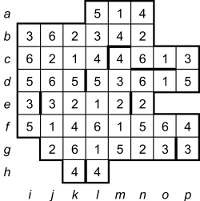The grid has 16 cells containing the digits 7 to 9 (and no zeroes), so the 8 nets of 6 cells must occupy all of the 48 cells containing 1 to 6. We’ll use letter coordinates to identify them here. We start by drawing bars to separate adjacent cells containing the same digit.

The 4 in cell hk must be connected to the 6 in gk but not fk (another 4) or gl (which would isolate the 4 in hl), so the net continues with the 2 in gj, the 1 in fj, the 5 in fi and the 3 in either ei or ej. If it uses the 3 in ej, the only connected set of cells containing 1 to 6, including the 3 in ei would be {ei, di, (ci or dj), cj, ck, cl} but neither of those shapes can be folded to make a cube. So the first net (A) is {hk, gk, gj, fj, fi, ei} in a zigzag shape.

From the 3 in cp, the only 5 it can reach without duplicating a digit is the one in dp, so they’re connected. If these cells are connected to the 1 in do, there’s no 4 that can be reached without passing over a duplicated digit, so we have {dp, cp, co, cn, bn} connected, needing a 4. They can’t take the 4 in cm because that would leave no way of separating the two 4s at the top into different nets; they can’t take the 4 in an because that would leave {al, am, bl, bm} connected, but a square of four cells can’t be folded into part of a cube. Thus the second net (B) is {dp, cp, co, cn, bn, bm}. The only possibility for the net C starting in the top row is then {an, am, al, bl, bk, bj}.

From the 3 in gp, net D must contain {gp, fp, fo, fn} and the 2 in either em or en (not gn because that would isolate the 3 in go). That forces the following cells to form net E: {go, gn, gm, gl, hl, fl}. From the 1 in do, net F must contain {do, dn, dm, dl, cm} and whichever of the 2s in {em, en} isn’t used by net D.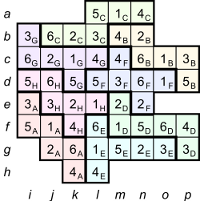For net G, the 3 in bi is connected to the 6 in ci; if they’re connected to the 5 in di, the net is forced into the same T-shape as net E, so net G must be {bi, ci, cj, ck, cl, dk}. That leaves net H enclosed as {di, dj, ej, ek, el, fk}.

If net D uses the 2 in en, that would make it the same shape as net G, rotated by 180 degrees. So net D is {gp, fp, fo, fn, fm, em}, net F is {cm, dl, dm, dn, do, en} and the partitioning is complete.

#### Stage 3: Assembling the dice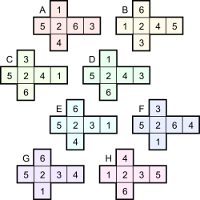At this stage it may be helpful to actually assemble the dice for physical manipulation. Here, we’ll start by reforming the nets into the same cross shape used for the larger cube, with the 5 and 2 in the corresponding places, where possible. Note that two of the dice, B and H, have 5 and 2 on opposite faces, not adjacent.

We’ll label the positions for dice in the larger cube S to Z, and the faces of each of these dice a to f, so, for example, the external faces showing 2 are {Sc, Tc, Uc, Vc}. The nets for the dice in the larger cube are shown here in the same layout as the rest, with the hidden (internal) faces shaded.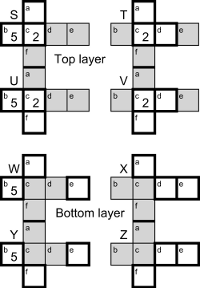In dice position T, the 5 can’t be on face Ta or Td (because they’re external faces, and the large cube has 5 on a different face), or on Tb (because that touches Sd internally, which can’t have 5 because dice S already has 5 on a different face); so the 5 is either on Tf or Te. Similarly, position V can have its 5 only on Va or Ve. Since Tf and Va are touching faces and must have the same digit, either (i) Te = Ve = 5, or (ii) Tf = Va = 5.

By similar logic, X and Z have only two places where the 5 could go, giving either Xf = Za = 5 or Xc = Zc = 5. Also, they can’t have 2 on any of their external faces (Xa, Xd, Xe, Zd, Ze, Zf) or on their top faces (Xc, Zc, because they touch the bottom faces of T and V, which have 2 on the top), so either Xb = Zb = 2 or Xf = Za = 2. For any combination of where the 2 and 5 can go on X and Z, they’re on adjacent faces, so neither dice B nor H can be at position X or Z.

##### Option (i):

If Te = Ve = 5 (which means Xc = Zc = 5, too), the dice at T and V have 2 and 5 on opposite faces, so they must be dice B and H. Matching up their {1, 3, 4, 6} faces for the touching faces Tf and Va, the only arrangements that show the same digit in the Td and Vd positions are (iii) T = H, V = B with Tf = Va = 1, Td = Vd = 6, and (iv) T = B, V = H with Tf = Va = 6, Td = Vd = 1. Both arrangements have 4 for the touching faces {Tb, Sd}, 3 for the external faces {Ta, Sa, Wa, Xa}, 3 for the touching {Vb, Ud} and 4 for the external {Vf, Uf, Yf, Zf}. Thus S has 524_ in the middle row and can only be dice C (5241, not D with 5243, because S has 3 on face Sa) and U has 523_ and must be dice E (5231, not G with 5234, because U has 4 on face Uf).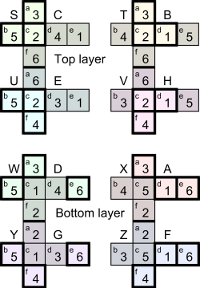Since the internal faces Se and Ue both have 1, their touching faces Wc and Yc must also have 1. We have the external faces identified for {2, 3, 4, 5}, so the bottom external face {We, Xe, Ye, Ze} must show 1 or 6. But We can’t be 1 because we have 1 at Wc, so the bottom face has 6 and the remaining external face {Td, Vd, Xd, Zd} has 1. That rules out option (iii) above, and we have T = B, V = H.

We now have {Xb, Xf} = {2, 4} in some order and {Za, Zb} = {2, 3} in some order, but Xf touches Za, so Xf = Za = 2, Xb = 4, Zb = 3. Orienting X and Z in the standard 5-2-cross formation, we can see that the dice in position X is A and the dice in position Z is F. The remaining faces of W and Y are easily filled in, and they match the remaining dice, W = D, Y = G. This is the first complete arrangement.

##### Option (ii):

If Tf = Va = 5, then the only positions for dice B and H (with 2 and 5 on opposite faces) are W and Y; thus Wd = Yd = 2 (and their touching faces, Xb and Zb, also have 2). Matching up the {1, 3, 4, 6} faces of B and H for the touching faces Wf and Ya, the only arrangements that show the same digit in the We and Ye positions are (v) W = B, Y = H with Tf = Va = 6, Td = Vd = 1, and (vi) W = H, Y = B with Tf = Va = 1, Td = Vd = 6. Both arrangements have 4 for the touching faces {Wc, Se}, 3 for the external faces {Wa, Sa, Ta, Xa}, 3 for the touching {Yc, Ue} and 4 for the external {Yf, Uf, Vf, Zf}. Thus S has 52_4 in the middle row and can only be dice F (5264, not G with 5234, because S has 3 on face Sa) and U has 52_3 and must be dice A (5263, not D with 5243, because U has 4 on face Uf).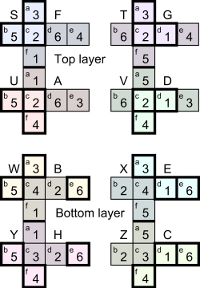Since the internal faces Sd and Ud both have 6, their touching faces Tb and Vb must also have 6. We have the external faces identified for {2, 3, 4, 5}, so the right external face {Td, Vd, Xd, Zd} must show 1 or 6. But Td can’t be 6 because we have 6 at Tb, so the right face has 1 and the remaining external face {We, Xe, Ye, Ze} has 6. That rules out option (vi) above, and we have W = B, Y = H.

Te = 4 completes the T position, which (using the 5-2-cross formation) we recognise as T = G. Ve = 3 completes the V position, which we recognise as V = D. The remaining faces of X and Z are easily filled in, and they match the remaining dice, X = E, Z = C. This is the second complete arrangement.

Both arrangements can be seen to have the same digits on the external faces, which in the 5-2-cross formation are 3-5216-4.